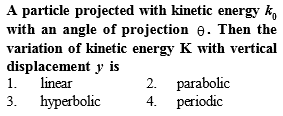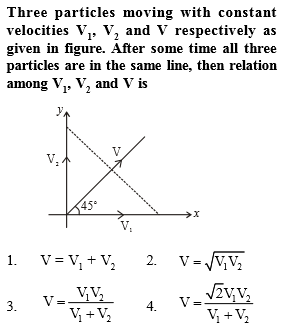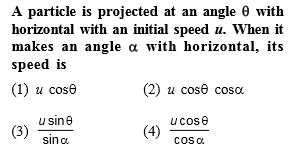Complete Question Bank + Test Series
Complete Question Bank

Difficulty Level: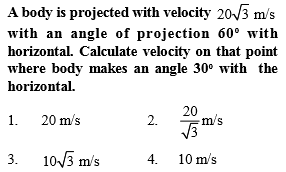Complete Question Bank + Test Series
Complete Question Bank

Difficulty Level:

A particle is moving with veocity $\stackrel{⇀}{\mathrm{V}}=k\left(y\stackrel{^}{i}+x\stackrel{^}{j}\right)$; where k is constant. The general equation for path is:

1. $\mathrm{y}={\mathrm{x}}^{2}+\mathrm{constant}$

2. ${\mathrm{y}}^{2}={\mathrm{x}}^{2}+\mathrm{constant}$

3. $\mathrm{y}=\mathrm{x}+\mathrm{constant}$

4. xy=constant

Complete Question Bank + Test Series
Complete Question Bank

Difficulty Level: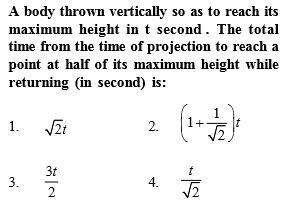Complete Question Bank + Test Series
Complete Question Bank

Difficulty Level: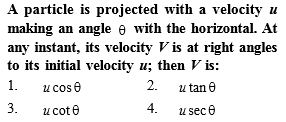Complete Question Bank + Test Series
Complete Question Bank

Difficulty Level: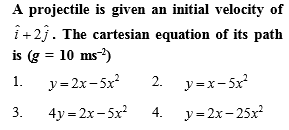Complete Question Bank + Test Series
Complete Question Bank

Difficulty Level: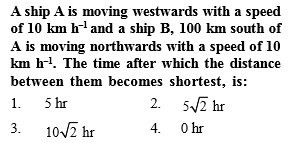Complete Question Bank + Test Series
Complete Question Bank

Difficulty Level: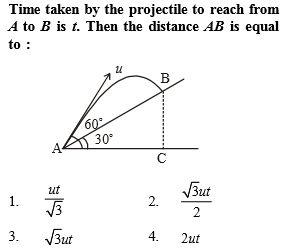Complete Question Bank + Test Series
Complete Question Bank

Difficulty Level: